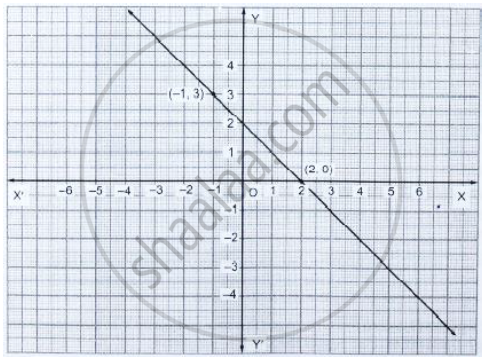# From the Choices Given Below, Choose the Equation Whose Graph is Given in Fig. Below. (I) Y = X + 2 (Ii) Y = X – 2 (Iii) Y = −X + 2 (Iv) X + 2y = 6 - Mathematics

From the choices given below, choose the equation whose graph is given in fig. below.
(i)  y = x + 2   (ii)  y = x – 2   (iii) y = −x + 2   (iv) x + 2y = 6[Hint: Clearly, (2, 0) and (−1, 3) satisfy the equation y = −x + 2]

#### Solution

Clearly ( 2, 0 ) and   (-1 , 3 ) satisfy the equation  y = -x + 2

∴ The equation whose graph is given by  y = - x + 2

Concept: Graph of a Linear Equation in Two Variables
Is there an error in this question or solution?

#### APPEARS IN

RD Sharma Mathematics for Class 9
Chapter 7 Linear Equations in Two Variables
Exercise 7.3 | Q 9

Share